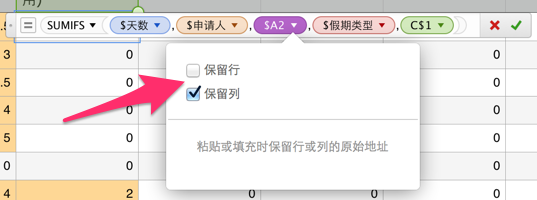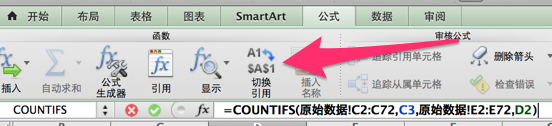# Execl函数中的固定单元格

Ghostzhang 发表于

 A B C 1 人员 休假类型 时间 2 userA 年假 2 3 userB 事假 0.5 4 userA 年假 1.5 2 userA 事假 1

 A B C D 1 人员 年假 事假 总次数 2 userA 3 userB

``````=COUNTIFS(原始数据!A:A,A1,原始数据!B:B,B1)
``````

``````=COUNTIFS(原始数据!A:A,A2,原始数据!B:B,B2)
``````

``````=COUNTIFS(原始数据!B:B,B1,原始数据!C:C,C1)
``````

``````=COUNTIFS(原始数据!A:A,A1,原始数据!B:B,B1) //B2
=COUNTIFS(原始数据!A:A,A2,原始数据!B:B,B1) //B3
=SUM(B2,B3) //B4
=COUNTIFS(原始数据!A:A,A1,原始数据!B:B,C1) //C2
=COUNTIFS(原始数据!A:A,A2,原始数据!B:B,C1) //C3
=SUM(C2,C3) //C4
``````windows版的没有找到，但功能是支持的，同样只需要在要保留的行或列前面加上一个美元符号 \$ 即可。于是只要这样：

``````=COUNTIFS(原始数据!\$A:\$A,\$A1,原始数据!\$B:\$B,B\$1)
``````